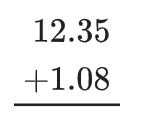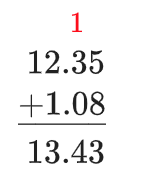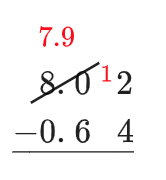### Home > CC1MN > Chapter 3 > Lesson 3.1.2 > Problem3-35

3-35.

Find the following sums or differences.

1. $12.35 + 1.08$

• Align the terms at their decimal points. Numbers arranged in 2 rows of 5 columns as follows: Top row: 1, 2, decimal, 3, 5. Bottom row: blank, 1, decimal point, 0, 8.

• Add each column, starting at the right. Since $5 + 8 > 10$, carry a 1 to the next column. Changes: Top row: 1 above the 3. Answer row: 1, 3, decimal point, 4, 3.

• $13.43$1. $8.02 − 0. 64$

Set up the problem by aligning the decimal points. Unlike in part (a), you will do subtraction for each column.

Notice that $2 < 4$, so you will need to ''borrow'' from the columns to the left. So a tenth was “borrowed” from 8.0 so that 4 would be subtracted from 12 instead of 2. That meant the 0.6 would be subtracted from 7.9.2. $568.38 − 134.21$

Use parts (a) and (b) for help.

3. $0.29 + 0.92$

Follow the steps from part (a). Remember that since $9 + 2 > 10$, you will need to carry a $1$ to the next column